## Introduction

$$\sum_{i=0}^{\infty} a_i x^i$$ Is light a wave or particle ? Can light be both a wave as well a particle ? How do we see ? How do we locate the position of objects so accurately in 3 dimensional space ?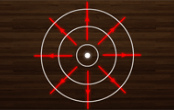Watch the video on Android App for free.

## LawsofReflection

What are the Laws of Reflection ? Does light reflect differently from plane and curved surfaces ?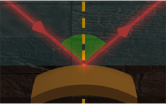Watch the video on Android App for free.

## Q1

A ray of light travelling in the direction is incident on a plane mirror. After reflection, it travels along the direction
Find the direction along which plane mirror is placed and the angle of incidence of the light ray.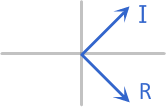Watch the video on Android App for free.

## RealVirtual

What is a Real object ? Does Real object mean that the object is actually present ? What is a Virtual object ? What is Real and Virtual image ?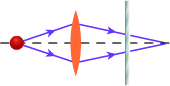Watch the video on Android App for free.

## Q2

Path of two light rays are traced through 3 optical systems, a Concave Lens, a Convex Lens and a Plane Mirror, as shown in figure. For each of the Concave Lens, Convex Lens and Plane Mirror, mark whether the object for it and the image formed by it is Real or Virtual.Watch the video on Android App for free.

## PlaneMirror

How does a Plane Mirror form an image of a point object ? Does the image formed by a plane mirror depend on the position of Observer ?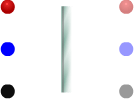Watch the video on Android App for free.

## Q3

i) An object is placed in front of a plane mirror. O, M and N are the three positions where the image of object may be seen. Observer A is able to see the image at position M. Mark the following statements as True / False
a) Observer B will see the image at position N
b) Observer C will see the image at position O
c) Both B and C will see the image at M
ii) Can we project the image formed by a plane mirror on to a screen? If yes, is the object real or virtual in that case ?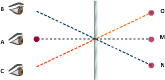Watch the video on Android App for free.

## Q4

A plane mirror M is arranged parallel to a wall W at some distance from it. The light produced by a point source S kept on the wall is reflected by the mirror and produces a patch of light on the wall. The mirror moves with velocity 'v' towards the wall. Which of the following statement(s) is/are correct?
a) The patch of light will move with speed v on the wall
b) The patch of light will not move on the wall
c) As the mirror comes closer, the patch of light will become larger
d) The size of the patch of light on the wall remains the sameWatch the video on Android App for free.

## PM-VelofImg-1

If the object is moving, how does its image in the plane mirror move ? What is the velocity of image formed by a plane mirror ?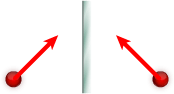Watch the video on Android App for free.

## PM-VelofImg-2

If both object and mirror are moving, how does the image move ? What is the velocity of image formed by a plane mirror ?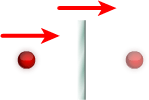Watch the video on Android App for free.

## Q5

i) An object and a plane mirror are moving towards each other, perpendicular to the plane of mirror, with velocities 2 m/s and 3 m/s respectively.
Find the velocity of the image
a) wrt Mirror
b) wrt Ground
c) wrt Object
ii) A plane mirror and an object are moving as shown in the figure. Find the velocity of the image
a) wrt Mirror
b) wrt Ground
c) wrt Object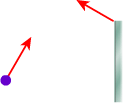Watch the video on Android App for free.

## Q6

A point object is kept in front of a stationery plane mirror. If the object performs SHM perpendicular to the plane of mirror with amplitude A, what will be the amplitude of SHM of its image ? If the object is kept stationery, but the plane mirror is doing SHM perpendicular to the plane of mirror with amplitude A, what will be the amplitude of SHM of the image of object ?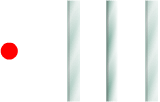Watch the video on Android App for free.

## Q7

For a fixed incident ray of light, if the mirror is rotated through an angle q ( about an axis in the plane of mirror ) as shown in figure, show that the reflected ray turns by an angle 2q in same direction.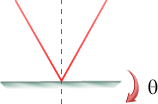Watch the video on Android App for free.

## Q8

A ray of light travels from a light source S to an observer after reflection from a plane mirror. If the source rotates in the clockwise direction by q, by what angle and in what direction must the mirror be rotated so that the light ray still strikes the observer?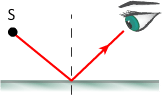Watch the video on Android App for free.

## ExtendedObj

How is the image of an extended object (non-point object) formed by a plane mirror ? Is the image distorted ?Watch the video on Android App for free.

## HeightofMirror

What is the minimum height of a plane mirror required to see the complete image of an object ? Does it depend on the position of object or mirror ?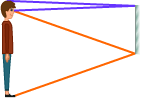Watch the video on Android App for free.

## Q9

A real point source is 5 cm away from a plane mirror, while the eye of an observer (pupil diameter 4 mm) is 10 cm away from the mirror. Assume that both source and eye are on the same line perpendicular to the mirror. Find the area of the mirror used to see the complete image of source.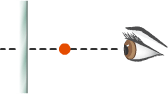Watch the video on Android App for free.

## ParallelMirror

How are multiple images formed by a set of plane mirrors placed parallel to each other ? Can we find the exact position of all the images formed ?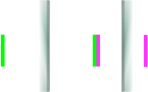Watch the video on Android App for free.

## Q10

Figure shows a point object placed between two parallel mirrors. Its distance from M1 is 6 cm and that from M2 is 4 cm. Find the position of images from the two mirrors considering first reflection on
a) mirror M1
b) mirror M2
c) Find the distance between the first image formed by M1 and the second image formed by M2.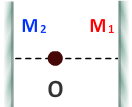Watch the video on Android App for free.

## InclinedMirror

How are multiple images formed by a set of plane mirrors inclined at an angle wrt to each other ? Can we find the exact position of all the images formed ?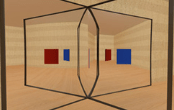Watch the video on Android App for free.

## Q11

Two mirror are placed perpendicular to each other. Find the number of images if the object is placed
a) on the angle bisector of mirrors
b) on a line making an angle of 30o with one of the mirrors. Locate the position of images in each case to justify the answer in above cases.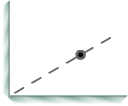Watch the video on Android App for free.

## Q12

Two plane mirrors are inclined at an angle of 120o to each other. Find the total number and location of images for the two positions of object shown in figure.Watch the video on Android App for free.

## Q13

Two mirrors are inclined at an angle of 30o. An object is placed making 10o with the mirror M1. Find the total number of images and the positions of all images formed by the mirrors.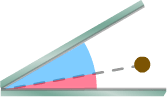Watch the video on Android App for free.

## SphericalMirror

What are Spherical Mirrors ? Does light reflect differently from Spherical mirrors ? Are Laws of Reflection valid for Spherical mirrors ?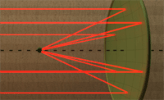Watch the video on Android App for free.

## OnAxis

Can we relate the position of Image wrt the position of Object for Spherical Mirrors ? Watch the video to see the Visual method developed by Nlytn which it much easier to visualize and remember the relation between the position of Image and Object without using any equations.Watch the video on Android App for free.

## OffAxis-1

Can we relate the Nature of Image wrt the position of Object for Concave Mirror ? Watch the video to see the Visual method developed by Nlytn which it much easier to visualize and remember the Nature of Image formed by the Concave Mirror without using any equations.Watch the video on Android App for free.

## OffAxis-2

Can we relate the Nature of Image wrt the position of Object for Convex Mirror ? Watch the video to see the Visual method developed by Nlytn which it much easier to visualize and remember the Nature of Image formed by the Convex Mirror without using any equations.Watch the video on Android App for free.

## Q14

Find the angle of incidence of the ray moving parallel to the principle axis of a Concave Mirror so that it will pass through the pole after reflection.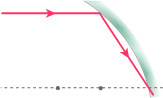Watch the video on Android App for free.

## Q15

Mark the following statements as True/False
a) For a spherical mirror, if object is on the left side of the focus, then its image will be on the right side of the focus .
b) Maximum distance of an image of a real object from a convex mirror is equal to the focal length of mirror.
c) For a real extended object, a Concave mirror can form a diminished virtual image
d) For a virtual extended object, a Convex mirror can form a diminished real imageWatch the video on Android App for free.

## Q16

a) A person looks into a spherical mirror. The size of erect image of his face is twice the actual size of his face. Is the mirror Concave or Convex ?
b) An image of a candle in a spherical mirror is found to be one third of its size. When the candle is shifted by some distance, then the image becomes half of its size. Find the nature of mirror if the image is given to be
i) erect
ii) inverted in the initial as well as final position
c) A person is looking at the image of his face in a spherical mirror. The image is erect. When he moves the mirror away from his face, the image is inverted. What type of mirror is he using ?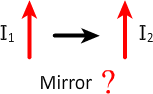Watch the video on Android App for free.

## Q17

Match the following :
 Column I Column II Column III Column IV Real object, Real image Concave Mirror Diminished Inverted Virtual object, Virtual image Convex Mirror Same Size Erect Real object, Virtual image Plane Mirror Enlarged Virtual object, Real image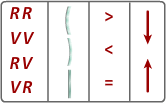Watch the video on Android App for free.

## SignConvention

Sign Convention for for working with Spherical Mirrors and for using Mirror Formula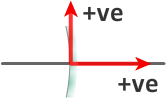Watch the video on Android App for free.

## MirrorFormula

Derivation, Explanation and Application of Mirror Formula. How can we relate the Mirror Formula to the Visual approach discussed in the previous videos ?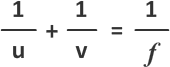Watch the video on Android App for free.

## Q18

i) Mirror formula is valid only for mirrors whose sizes are very small compared to their radii of curvature. True / False
ii) A beam of parallel incident rays is being reflected as a parallel beam by a mirror as shown in figure. The Mirror can be a
a) plane mirror
b) convex mirror
c) concave mirror
d) may be any spherical mirror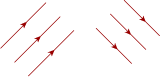Watch the video on Android App for free.

## Q19

a) Converging rays fall on a convex spherical mirror with radius of curvature 60 cm so that the reflected rays converge on the principal axis of the mirror at a distance of 30 cm from the pole of mirror. If there was no mirror, what will be the distance of the intersection point of the incident rays from the current position of pole of mirror.
b) Converging rays are incident on a convex spherical mirror so that their extensions intersect 30 cm behind the mirror on the optical axis. The reflected rays from a diverging beam so that their extension intersect the optical axis 1.2 m from the mirror. Find the focal length of the mirror.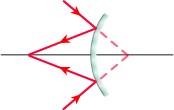Watch the video on Android App for free.

## Q20

A student performed the experiment of determination of focal length of a concave mirror which is known to be 24 cm. The 5 sets of ( u, v ) values recorded by the student (in cm) are : (42, 56), (48, 48) (60,40), (66, 33), (78,39). The data set(s) which is (are) incorrect, is/are a) (42, 56)
b) (48, 48)
c) (66, 33)
d) (78,39)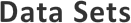Watch the video on Android App for free.

## Q21

An extended object is placed in front of a concave mirror of focal length 15 cm. Find the position and nature of the image, if the position of object is
a) -40 cm
b) -20 cm
c) -10 cm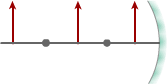Watch the video on Android App for free.

## Magnification

Do Spherical Mirrors cause magnification ? Derivation, Explanation and Application of equation of Magnification due to Spherical Mirrors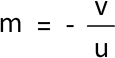Watch the video on Android App for free.

## Q22

A spherical mirror has a focal length f. Find the general expression of the two positions of the object for which the image size is
a) three times the size of object
b) 1/3 times the size of object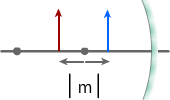Watch the video on Android App for free.

## Q23

A concave mirror gives a real image magnified 4 times. When the object is moved 3 cm the magnification of the real image is 3 times. Find the focal length of mirror. The image of a real object in a convex mirror is 4 cm from the mirror. If the mirror has a radius of curvature of 24 cm, find the position of object and magnification.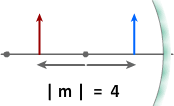Watch the video on Android App for free.

## Q24

A particle revolves in a circle of radius 5 cm (clockwise as seen from a point behind the object and looking towards the mirror) at a distance of 15 cm from a convex mirror and completes one revolution in 5 sec. The axis of the circle and the principal axis of the mirror coincide. The radius of curvature of the mirror is 20 cm. Find the direction of revolution of the image of the particle and its speed.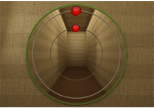Watch the video on Android App for free.

## Q25

A point object moves on a straight line which makes an angle 'q' with the principal axis, as shown in figure. Show that image also moves in straight line making the same angle q with principle axis.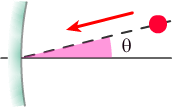Watch the video on Android App for free.

## LongitudinalMagnification

What is Longitudinal Magnification ? Is the equation of Longitudinal Magnification an approximation ? What is the criteria for the equation of Longitudinal magnification to be valid ? See the derivation and discussion which cannot be found anywhere else !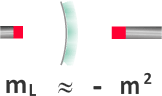Watch the video on Android App for free.

## Q26

An object is placed along the axis of a concave mirror of focal length 10 cm. Closer end of the object is at a distance of 30 cm from the mirror. Find the length of the image,
a) if length of object is 1 mm
b) if length of object is 5 cm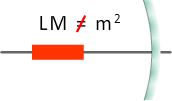Watch the video on Android App for free.

## Q27

A point object is placed 60 cm from the pole of a concave mirror of focal length 10 cm on the principal axis. If the object is shifted 1 mm towards the mirror along principal axis, find the shift in image. What will be the shift in image, if the object is placed at 10.5 cm from the mirror.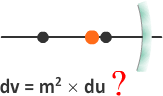Watch the video on Android App for free.

## SM-VelofImg

If the object is moving infront of a Concave/Convex Mirror, how does its image move ? Can we find the velocity of image ? Is the velocity of image wrt Mirror or wrt Ground ? What if the Mirror is also moving ?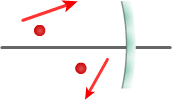Watch the video on Android App for free.

## Q28

If the object is moving infront of a Concave/Convex Mirror, how does its image move ?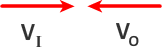Watch the video on Android App for free.

## Q29

A mirror of focal length 30 cm and an object which is placed at a distance of 15 cm are both moving with velocities 10 cms-1 and 6 cms-1 as shown in the figure. Find
a) the velocity of image wrt mirror
b) the velocity of image wrt ground
c) the velocity of separation of object and image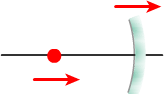Watch the video on Android App for free.

## Q30

i) A point object is moving perpendicular to the principle axis. Find the relation between the speed of object and speed of its image wrt mirror interms of magnification ii) A point object is at the center of curvature of a concave mirror. The mirror starts to move with a constant speed of +V perpendicular to the principal axis. Find the velocity of the image
a) wrt Mirror
b) wrt Ground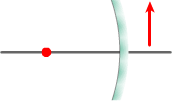Watch the video on Android App for free.

## Q31

An object is traveling toward a concave mirror of focal length 10 cm. It moves with a velocity of 0.1 m/s parallel to the principal axis and at a height of 1 cm from the principal axis. When it is at a distance of 15 cm from the mirror, find the velocity of image in x and y direction.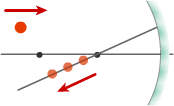Watch the video on Android App for free.

## 1

In the 2 arrangements of a pair of plane mirrors shown below, the image of the extended object as seen by the observer can be
a) real and inverted
b) real and erect
c) virtual and inverted
d) virtual and erectWatch the video on Android App for free.

## 2

Two plane mirrors A and B are aligned parallel to each other, as shown in the figure. A light ray is incident at an angle 30o at a point just inside one end of A. The plane of incidence coincides with the plane of the figure. The maximum number of times the ray undergoes reflections ( including the first one ) before it emerges out is
a) 28
b) 31
c) 32
d) 34Watch the video on Android App for free.

## 3

a) A point source of light S, placed at a distance L in front of the center of a plane mirror of width d, hangs vertically on a wall. A man walks in front of the mirror along a line parallel to the mirror at a distance 2L from it, as shown. Find the distance over which he can see the image of the light source in the mirror.
b) A mirror of length 2d is kept in front of a road and a point object is kept at a distance d from the mirror, as in above question. An observer is able to see the image for a distance 10 d while moving along the road. What is the distance between the road and the mirror?Watch the video on Android App for free.

## 4

A ray of light makes an angle of 10o with the horizontal and strikes a plane mirror which is inclined at an angle q with the horizontal. For what value of q will the reflected ray become vertical ?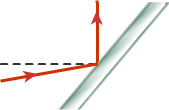Watch the video on Android App for free.

## 5

A light ray is incident on a plane mirror. The mirror is rotated with angular speed w. The light reflected by the mirror falls on a circular wall surrounding the entire setup and the light forms a spot on the wall. What will be the angular speed of the spot on the wall ? A light ray is incident on a plane mirror which is rotated with angular speed w. The light reflected by the mirror falls on a straight wall as shown in the figure. Find the expression of the speed of spot on the wall when the angle of incidence is q. Incident ray is fixed and makes an angle f with the plane of wall.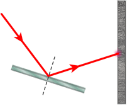Watch the video on Android App for free.

## 6

A plane mirror, an object O and a viewer V are moving as shown in the figure. a) What is the velocity of image of object O wrt Mirror ? b) What is the velocity of image of object O as observed by viewer V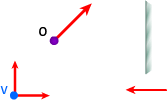Watch the video on Android App for free.

## 7

i) A man is walking under an inclined mirror at a constant velocity V m/s along the x-axis. If the mirror is inclined at an angle q with the horizontal. What is the
a) Speed of Image wrt Mirror and wrt Object
b) Velocity of the image wrt Ground, wrt Mirror and wrt Object in terms of x and y components
ii) A plane mirror is placed at origin parallel to y-axis, facing the positive x-axis. An object is moving with a velocity of ( 2i + 2j ) m/s. Find the relative velocity of the image with respect to the object.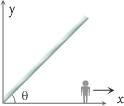Watch the video on Android App for free.

## 8

Two plane mirrors are placed at angle q. A ray of light is incident on the first mirror and after reflection it strikes the second mirror and is again reflected from the second mirror, as shown in figure.
a) Find the relation between the angle q and the angle of incidence on the first mirror so that the ray 3 is parallel to the first mirror.
b) If ray 1 is parallel to the second mirror and ray 3 is parallel to the first mirror, find the angle between the two mirrors.
c) If ray 1 makes an angle of 60o with first mirror and ray 3 is parallel to the first mirror, find the angle between the mirrors.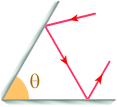Watch the video on Android App for free.

## 9

a) Two plane mirrors are placed at an angle q as shown in figure. If the incident ray strikes the first mirror such that the angle of incidence is a, find the angle of deviation of incident ray after the ray is reflected from the second mirror.
b) Find the angle of inclination between two plane mirror so that the incident ray and the reflected ray from the two mirrors are always parallel to each other, irrespective of the angle of incidence.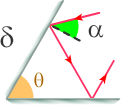Watch the video on Android App for free.

## 10

In an experiment to determine the focal length of a concave mirror, a student places an object A on the principal axis at a distance 'x' from the pole P of mirror. The student looks at the object and its inverted image from a distance keeping his/her eye in the line with PA. When the student shifts his/her eye towards left, the image appears to the right of the object pin. Then a) x < f b) f < x < 2f c) x = 2f d) x > 2f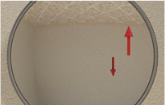Watch the video on Android App for free.

## 11

Sun subtends an angle of 1/2o at the pole of a concave mirror, which has a radius of curvature of 10 m. Find the diameter of the image of sun formed by the concave mirror.Watch the video on Android App for free.

## 12

The inner surface of a cone coated by a reflecting layer forms a conical mirror. A thin incandescent filament is stretched in the cone along its axis. Determine the minimum angle q of the cone for which the rays emitted by the filament will be reflected from the conical surface not more than once.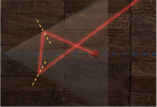Watch the video on Android App for free.

## 13

The distance between a real object and its image in a convex mirror of focal length 12 cm is 32 cm. Find the height of image if the height of the object is 1 cm.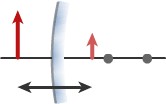Watch the video on Android App for free.

## 14

When an object is placed at a distance of 25 cm from a concave mirror, the magnification is m1. The magnification becomes m2 when the object is moved 15 cm farther away with respect to the earlier position. If m1/m2 = 4, then find the focal length of the mirror.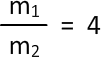Watch the video on Android App for free.

## 15

If distance between an object and its image being equal to l, the transverse magnification is = b Determine the focal length of a concave mirror.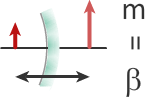Watch the video on Android App for free.

## 16

The image of an object placed on the principal axis of a concave mirror of focal length 12 cm is formed at a point which is 10 cm more distance from the mirror than the object. Find the magnification of the image.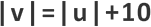Watch the video on Android App for free.

## 17

A square ABCD of side 10 cm is kept in front of the concave mirror as shown in fig. The focal length of the mirror is 25 cm. Find the location and magnification of sides BC and AD and draw the shape of image of square ABCD, if side BC is at a distance of
a) 50 cm from pole
a) 30 cm from pole
a) 10 cm from pole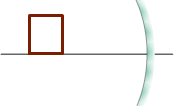Watch the video on Android App for free.

## 18

A cube of side 2 m is placed in front of a concave mirror of focal length 1 m with its face A at a distance of 3 m and face B at a distance of 5 m from the mirror. Find the heights of images of A and B and the distance between the images of faces A and B.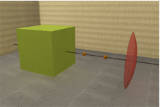Watch the video on Android App for free.

## 19

A concave mirror forms a real image, three times larger than the object on a screen. The object and screen are moved until the image becomes twice the size of the object. If the shift in the position of object is 6 cm, find the shift in the position of screen.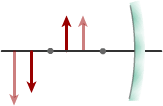Watch the video on Android App for free.

## 20

A mirror is broken into two parts and these parts are separated by a distance of 2 cm as shown in figure. Find the location of the images formed by the two parts of mirror. The object is midway between the two principal axes.Watch the video on Android App for free.

## 21

An object of length 5 cm is placed infront of a convex mirror as shown in figure. Find the length of image.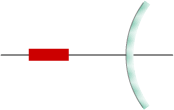Watch the video on Android App for free.

## 22

a) A short linear object of length b lies along the axis of a concave mirror of focal f at a distance u from the pole. What is the size of image? b) if the object begins to move with speed Vo , what will be the speed of its image? Take u - f >> bWatch the video on Android App for free.

## 23

An object is moving towards a concave mirror of radius of curvature R = 30 cm along its principal axis with a constant speed of V = 18 cm/sec.
a) Find the speed of the image when the object is at a distance of 60 cm from the pole of the mirror.
b) When will the speed of object and image be equal ?
c) In which region of principle axis will the object be, for the speed of image to be more than the speed of object ?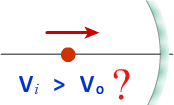Watch the video on Android App for free.

## 24

Image of an object approaching a convex mirror of radius of curvature 20 m along its optical axis is observed to move from 25/3 m to 50/7 m in 30 s. What is the speed of the object in km/h ?Watch the video on Android App for free.

## 25

A point mass is placed on the principal axis of a concave mirror of radius of curvature R = 20 cm at a distance of 60 cm from the pole of the mirror. The point mass is executing simple harmonic motion along the principle axis. Find the amplitude of oscillation of the image formed by the mirror, if
a) amplitude of oscillation is 1 cm
b) amplitude of oscillation is 10 cmWatch the video on Android App for free.

## 26

A thin rod of length f/3 is placed along the optic axis of a concave mirror of focal length f such that its image which is real and elongated, just touches the rod. Find the longitudinal magnification of rod.Watch the video on Android App for free.

## 27

A linear object AB is placed along the axis of a concave mirror with focal length = f. The object is moving with speed V. The speed of the image of both points A and B is 4V. Find the length of object in terms of the focal length of mirror.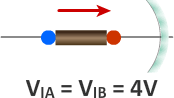Watch the video on Android App for free.

## 28

A ray of light strikes at the edge of a spherical concave mirror as seen in the figure. After reflection it crosses the optical axis at A at a distance FA from the focus.
a) Calculate the distances FA and FB in terms of the focus f and angle q.
b) Calculate the distances FA in terms of the radius of curvature R and distance h of the ray from principle axis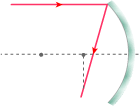Watch the video on Android App for free.

## 29

A concave spherical mirror with an aperture diameter of 60 cm has radius of curvature of 50 cm. Calculate the longitudinal spherical aberration of the edge ray moving parallel to the principal axis. Also calculate the maximum height 'h' from the optical axis, where the rays should strike the mirror, so that the longitudinal spherical aberration does not exceed 2 % of the focal length.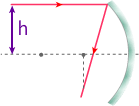Watch the video on Android App for free.

## 30

An object is placed between a plane mirror and a concave mirror of focal length 15 cm as shown in figure. Find the positions of the images after three successive reflections, considering the first reflection
a) on plane mirror
b) on concave mirror Repeat the above question, if the focal length of concave mirror is 20 cm.Watch the video on Android App for free.

## 31

A plane mirror is placed at a distance of 50 cm from a concave mirror of focal length 16 cm.Where should an object be placed between the mirrors so that its virtual image in the plane mirror coincides with the real image in concave mirror? What is the ratio of the sizes of the two images? An object is placed in front of a convex mirror at a distance of 50 cm. A plane mirror is introduced covering the lower half of the convex mirror. If the distance between the object and the plane mirror is 30 cm, it is found that there is no parallax between the images formed by the two mirrors. What is radius of curvature of the convex mirror?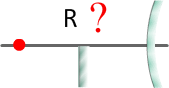Watch the video on Android App for free.

## 32

A source S is kept on the principal axis of a concave mirror of focal length f = 20 cm at a distance of 30 cm from its pole. Find the distance in front of the concave mirror where plane mirror should be placed so that the ray after being reflected first at the concave mirror and then by the plane mirror return to the point S. Repeat the above question, if the ray is first reflected at the plane mirror and then at the concave mirror.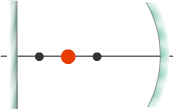Watch the video on Android App for free.

## 33

An object is placed 20 cm from the surface of a convex mirror and a plane mirror is placed somewhere between them so that the virtual images in the two mirrors coincide. If the plane mirror is 12 cm away from the object, what is the focal length of the convex mirror ?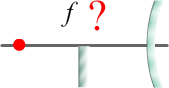Watch the video on Android App for free.

## 34

An object is placed midway between a concave mirror and a convex mirror both of focal length f. The distance between the two mirrors is 6f. Locate the position of image after 1st and 2nd reflection, if a) the ray is first incident on the concave mirror and then on the convex mirror b) the ray is first incident on the convex mirror and then on the concave mirror Also trace the rays to locate the position of images in both cases.Watch the video on Android App for free.

## 35

Two concave mirrors are placed 40 cm apart and are facing each other. A point object lies between them at a distance of 12 cm from the mirror of focal length 10 cm. The other mirror has a focal length of 20 cm. Find the location of final image formed after two reflections - first at the mirror nearer to the object and second at the other mirror. A point object is placed somewhere between two concave mirrors having focal lengths as shown in figure. Find the value of d for which only one image is formed.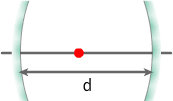Watch the video on Android App for free.

## 36

A convex and a concave mirror of focal lengths 50 and 40 cm respectively are placed coaxially and facing each other at a distance of 2 m. A circle of radius 8 mm is drawn on the convex mirror with its pole as centre. Find the position and radius of the image after two reflections, first at concave and then at convex mirror.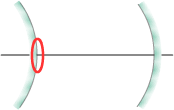Watch the video on Android App for free.

## 37

A concave mirror of focal length 10 cm and a convex mirror of focal length 15 cm are placed facing each other 40 cm apart. An object of height 2 cm is placed between the mirrors, on their common axis and 15 cm from the concave mirror. Find the position, size and nature of the image produced by 2 successive reflections, first at the concave mirror and then at the convex mirror.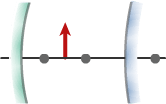Watch the video on Android App for free.

## 38

In situation shown below Convex mirror has a focal length of 10 cm. Object moves with velocity 10 cm/s and mirror moves the velocity 2 cm/s as shown. What is the velocity of image
a) wrt Mirror
b) wrt Ground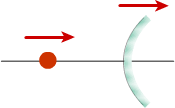Watch the video on Android App for free.

## 39

A car is fitted with a convex mirror of focal length 3 m. A second car 2 m broad and 1.6 m high is 6 m behind the first car. Find
a) the position of the second car as seen in the mirror of the first car
b) the breadth and height of the second car seen in the mirror of the first car
c) if the second car is overtaking at a relative speed of 9 m/s, what is the speed of image of second car w.r.t first car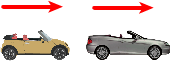Watch the video on Android App for free.

## 40

A gun of mass m2 fires a bullet of mass m1 with a horizontal speed V1. The gun is fitted with a concave mirror of focal length f facing the fired bullet. Find
a) the speed of image of bullet wrt mirror just after the gun was fired
b) the speed of separation of the bullet and its image just after the gun was fired. Express your answer in terms of m1 , m2 and V1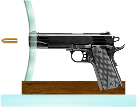Watch the video on Android App for free.

## 41

A small block of mass M and a concave mirror of radius R fitted with a stand lie on a smooth horizontal table with a separation d between them. The mirror together with its stand has a mass m. The block is pushed at t = 0 towards the mirror so that it starts moving towards the mirror at a constant speed V and collides with it. The collision is perfectly elastic. Find the velocity of the image in terms of d, R, m, M and V, a) at a time t < d / V b) at a time t > d / VWatch the video on Android App for free.

## 42

Consider the situation shown in figure. The elevator is going up with an acceleration of 2 m/s2 and the focal length of the convex mirror is 12 cm. The system is released from rest ( with respect to the elevator ) at t = 0 when the distance of block B from the mirror is 42 cm. Find the speed of image of block B wrt mirror at t = 0.2 s. Take g = 10 ms-2.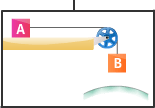Watch the video on Android App for free.

## 43

Two concave mirrors of equal radii of curvature R are fixed on a stand facing opposite direction. The whole system has a mass m and is kept on a frictionless horizontal surface as shown in figure. Two block A and B, each of mass m, are placed on the two sides of the mirrors at a distance 2R. Block A moves towards the mirror at a speed V. All collisions are given to be elastic. Taking the original position of the mirrors-stand system to be x = 0 and X- axis along AB, find
a) the position of the image of A at t = R/V
b) the position of the image of A and B at t = 3R/V
c) the position of the image of B at t = 5R/VWatch the video on Android App for free.